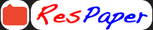Trending ▼   ResFinder# ICSE Class X Prelims 2021 : Computer Applications (St. Joseph's Convent School (SJCS), Panchgani)

2 pages, 25 questions, 6 questions with responses, 6 total responses,20Hitesh Dave +Fave Message ProfileTimelineUploads
 Home > dhl163 >   F Also featured on: School Page icseFormatting page ...

Class : X Date- 11/12/ 2020 St. Joseph s Convent School, Panchgani. Sub: Computer Applications Preliminary Examination Time: 2 Hrs. Marks: 100 Attempt all questions of Section A and any 4 questions of Section B. Section A (40 Marks) Q.1 ] a) Define Object with an example. b) Differentiate between Class variable and Instance variable. c) Write the java expression for: tan 1 x e 2 x d) Differentiate between operand and operator. e) What is polymorphism? Define the way of implementing polymorphism.  Q.2 ] a) State one similarity and one difference between = = and equals(). b) What are the types of casting shown by the following examples? (i) double x = 15.2; int y= (int) x; (ii) int x = 12; long y =x; c) Rewrite the following using switch-case.  char x; int m=0; if( x== a ) m=m+1; if(x== b ) m=m+2; d) What is the Dangling else problem in nested if-else construct. e) Rewrite using switch . . . case . . statement d = (m = = 1) ? 5 : 25 ; Q 3] a) What is an empty loop? Give an example. b) State the output of:   for(int i = 1; i<=6; i + +) { System.out.print( i + ); if( i % 2 = = 0) continue; System.out.println( ); } c) Name the jump statement that: (i) moves to the next iteration, while skipping the remaining code in the current iteration. (ii) enforce the early termination of a loop.  d) Re-write the following using for loop: int i=1, f=1; do { f= f*i; i++; } while( i< = 10); System.out.println( f ); e) Differentiate between base type and subscript type of an array.  f) if( a> 10 || b> 10) r = a* 2; else r = b* 3; What will be the value of r if : (i) a=100 b=90 (ii) a=10 b=10 (iii) a=25 b=10 g) State the output : Given: String x[ ] = { SJC , HIGH , SCHOOL }; 1) System.out.println( x.charAt(2) ); 2) System.out.println( x.lastIndexof ( O ) ); 3) System.out.println( x.substring(0, 1) + x.substring(1) );Formatting page ...

Top Contributors
to this ResPaperGrade10 GMA(3)Cbox(1)Shreya Bhandari(1)ResPaper Admins(1)